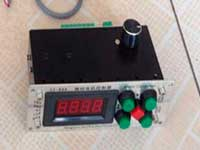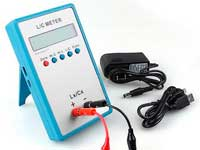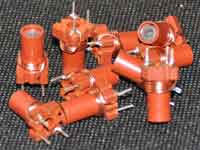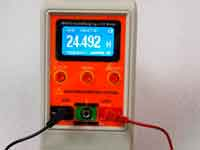Ferrite rod core inductance - 3.7 out of 5 based on 6 votes
Rating 3.67 (6 Votes)

Ferrite rod core inductance.Unlike a toroidal inductor on a ferrite ring, the magnetic flux of the coil on the ferrite rod is not fitting completely within the ferrite and each magnetic field line goes through the ferrite core and through the air, so the calculation of such coil is more complex. The inductance depends on:

• ferrite rod magnetic permeability and its sizes;
• the dimensions of the coil;
• the mutual ratio of sizes of the coil and rod;
• the position of the coil relative to the center of the rod.

Calculation of the coil inductance on a ferrite rod is based on the determination of the relative effective permeability of the rod. In other words, we need to determine how the inductance of the air-core coil will be increased if inside it to insert a ferrite rod. The basic formula looks like this:

 μe = Lf / Lair = (1 + x) / (1 / k + x / μfe ) 

,where Lf / Lair is the ratio of the inductance of the coil with the ferrite to the inductance of the same coil without ferrite and the factors xk & μfe are calculated according to the following algorithm:

1. l' = lc + 0.45 dc;
2. φ_φmax ≈ 1 / [ 1 + { ( ( lf - lc ) / df )1.4 } / ( 5 μ ) ];
3. Canf = 0.5 π ε0 ( lf - lc ) / [ ln { 2 ( lf + df) / df } - 1 ];
4. k = [ (φ_φmax Canf / ε0 ) + 2 df ] / 2 dc
5. x = 5.1 [ l' / dc ] / [1+ 2.8 ( dc / l' )];
6. μfe = ( μ -1) ( df /dc)2 +1;

where ε0 = 8,8542*10-12 F/m is the electric constant, μ is the initial magnetic permeability of the rod material. The dimensions in meters, see signs on the picture:A bit of theory explaining the algorithm:

• We can assume that the air core coil has a magnetic circuit consisting of two parts. Outside the coil and inside of it. They differ in the density of magnetic field lines and magnetic reluctance. If the magnetic reluctance of the inner part of the magnetic circuit is higher than the outer part, then the using of ferrite reduces the reluctance and has the effect to increase the inductance. The ratio of the magnetic reluctance of the two paths of the magnetic circuit of the air-core coil is marked in the basic formula  as x and it is computed in the 5th step of the algorithm..
• Factor μfe considers the case when the winding is not tight adjoined to the core, i.e. when there is a radial gap between the core and the coil.
• Factor Canf takes into account the influence of parts of the rod, which protrude from the coil. These parts reduce the magnetic reluctance of the outer part of the magnetic circuit and also increase the inductance.
• Factor φ_φmax shows the ratio of the actual magnetic flux in the rod with finite permeability to that with infinite permeability. This parameter, along with parameter Canf is used to calculate the factor k of the basic equation 

The shift of the coil relative to the rod reduces the inductance of the coil, this circumstance is taken into account using the correction factor K:

 K = -440.9943706*sh8+1318.707293*sh7 -1604.5491034*sh6+1021.078226*sh5 -363.8218957*sh4+71.6178135*sh3 -7.6027344*sh2+0.3013663*sh+0.995 

,where

• sh - relative offset = offset s divided by half the length of the core [sh = s / ( lf / 2 )].

This formula is obtained by regression analysis and is valid for s = 0,05..0,75.

In the end, the inductance of the coil on the ferrite rod is determined by the following formula:

 L(μH) = μe Lair*K 

The inductance of the air-core coil Lair is calculated according to the algorithm of a single layer coil calculation given the winding pitch. The length of the winding can be found by the following formula:

 lc = dw  + N * p 

,where

• dw - the wire diameter.
• p - the winding pitch.
• N - number of turns.

The calculating algorithm has the following limitations:

• The winding pitch cannot exceed the doubled wire diameter;
• The coil diameter cannot be greater than the doubled diameter of the rod;
• The winding length should be less than 3/4 length of the rod;
• When the coil is shifting it should not reach the edge of the core 1/8 of its length;
• The initial magnetic permeability of the rod should be greater than 100;

The calculation is based on the work of Alan Payne G3RBJ (see link below). The calculation is going by the formulas ,,  and  by an iteration method. The calculation is implemented in the plug-in version 12.2 and higher.

In addition, you can use the online calculator of the coil on a ferrite rod.References:

Special thanks to Andrey V. Cainov for constructive assistance and co-authorship in the development of methods of the calculation.

Сomments from anonymous guests are enabled with moderation.

Coil Winding MachineUS \$187.53
The system consists of individual components including a controller, a stepping motor, a foot switch, power adapter and output shaft. This design makes it very easy for you to integrate the system into your own project.

LC Meter LC200AUS \$37.99
Measuring Capacitance Range:
0.01 pF - 100mF
Measuring Inductance Range:
0.001 uH - 100H
Measurement accuracy:1%.

RF InductorsUS \$6.43
Variable Ferrite Core Inductor coil set 3.5Turns 25-100MHZ

Auto Ranging LCR MeterUS \$63.07
Meter can measure 0.00pF to 100.00mF capacitance, 0.000uH to 100.00H inductance and 0.00? to 10.000M? resistance. Very suitable for small value capacitor and inductor measurement.